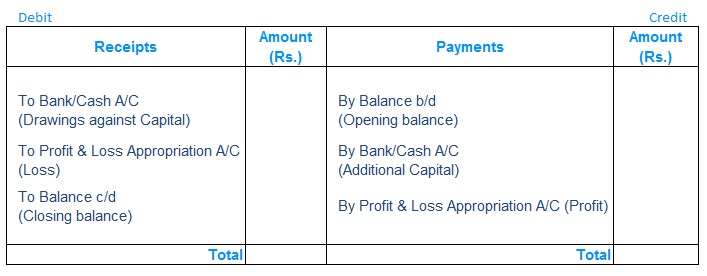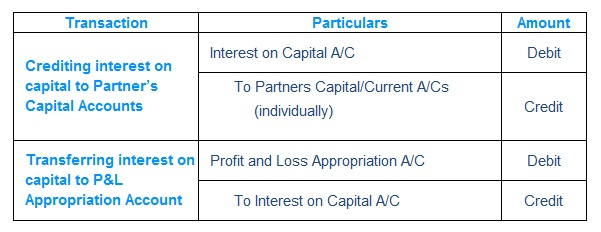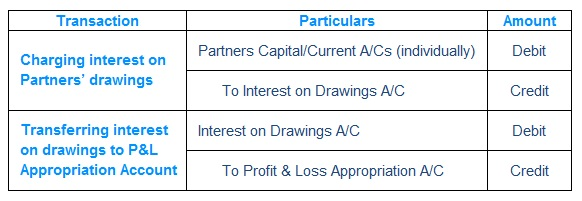# Partnership Fundamentals Notes2

## Partnership - Fundamentals

#### II.   INTEREST ON CAPITAL AND DRAWINGS

##### A. Interest on Capital
• Interest is computed on time proportion basis, number of days capital deployed in Firm
• Interest is computed only on Opening Capital, Additional Capital & drawings against capital
• Interest is not computed on Profit/Loss
• Interest on Capital= Amount of capital X Rate of Interest X Time
• If only Closing Capital is given, interest cannot be computed on Closing capital
• We need to find out Opening Capital and capital additions/deletions
• If Opening Capital is not given prepare Capital A/C or use formula
• Opening Capital=Closing Capital + Loss + Drawings- Profit – Additional Capital
• Prepare Ledger Account as per the below format
##### For the year ended …………………….##### Journal Entry##### B. Interest on Drawings
• Simple Method – Interest is computed for each drawing separately using simple interest formula:
•  Product Method – Interest is computed for each drawing separately using simple interest formula:

Product = Each Drawing x No. of Months till Financial year end

• Two prerequisites / condition of using this method
• Uniform/Similar time intervals…monthly, quarterly, half-yearly etc.
• Uniform/Similar amounts on each interval say 1000, 5000, 10000 etc.
##### Journal Entryerror: Content is protected !!